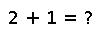# hessfact

``````..  hessfact(A)

Compute the Hessenberg decomposition of ``A`` and return a ``Hessenberg`` object. If ``F`` is the factorization object, the unitary matrix can be accessed with ``F[:Q]`` and the Hessenberg matrix with ``F[:H]``. When ``Q`` is extracted, the resulting type is the ``HessenbergQ`` object, and may be converted to a regular matrix with :func:`full`.``````

## Examples

Checking you are not a robot: